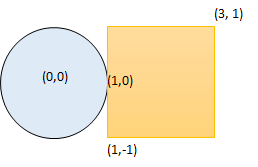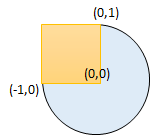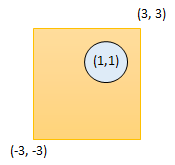# GeetCode Hub

Given a circle represented as (`radius`, `x_center`, `y_center`) and an axis-aligned rectangle represented as (`x1`, `y1`, `x2`, `y2`), where (`x1`, `y1`) are the coordinates of the bottom-left corner, and (`x2`, `y2`) are the coordinates of the top-right corner of the rectangle.

Return True if the circle and rectangle are overlapped otherwise return False.

In other words, check if there are any point (xi, yi) such that belongs to the circle and the rectangle at the same time.

Example 1:```Input: radius = 1, x_center = 0, y_center = 0, x1 = 1, y1 = -1, x2 = 3, y2 = 1
Output: true
Explanation: Circle and rectangle share the point (1,0)
```

Example 2:```Input: radius = 1, x_center = 0, y_center = 0, x1 = -1, y1 = 0, x2 = 0, y2 = 1
Output: true
```

Example 3:```Input: radius = 1, x_center = 1, y_center = 1, x1 = -3, y1 = -3, x2 = 3, y2 = 3
Output: true
```

Example 4:

```Input: radius = 1, x_center = 1, y_center = 1, x1 = 1, y1 = -3, x2 = 2, y2 = -1
Output: false
```

Constraints:

• `1 <= radius <= 2000`
• `-10^4 <= x_center, y_center, x1, y1, x2, y2 <= 10^4`
• `x1 < x2`
• `y1 < y2`

class Solution { public boolean checkOverlap(int radius, int x_center, int y_center, int x1, int y1, int x2, int y2) { } }